## Floquet Analysis

Given a system of periodic Ordinary Differential Equations of the form(1)

the solution can be written as a linear combination of functions of the form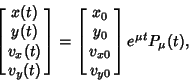(2)

where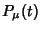is a function periodic with the same periodas the equations themselves. Given an Ordinary Differential Equation of the form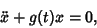(3)

where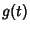is periodic with period, the ODE has a pair of independent solutions given by the Real and Imaginary Parts of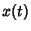(4)(5)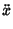(6)

Plugging these into (3) gives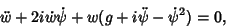(7)

so the Real and Imaginary Parts are(8)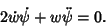(9)

From (9),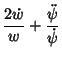(10)

Integrating gives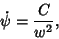(11)

whereis a constant which must equal 1, sois given by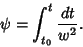(12)

The Real solution is then(13)

so(14)

and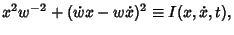(15)

which is an integral of motion. Therefore, although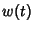is not explicitly known, an integralalways exists. Plugging (10) into (8) gives(16)

which, however, is not any easier to solve than (3).

References

Abramowitz, M. and Stegun, C. A. (Eds.). Handbook of Mathematical Functions with Formulas, Graphs, and Mathematical Tables, 9th printing. New York: Dover, p. 727, 1972.

Binney, J. and Tremaine, S. Galactic Dynamics. Princeton, NJ: Princeton University Press, p. 175, 1987.

Lichtenberg, A. and Lieberman, M. Regular and Stochastic Motion. New York: Springer-Verlag, p. 32, 1983.

Margenau, H. and Murphy, G. M. The Mathematics of Physics and Chemistry, 2 vols. Princeton, NJ: Van Nostrand, 1956-64.

Morse, P. M. and Feshbach, H. Methods of Theoretical Physics, Part I. New York: McGraw-Hill, pp. 556-557, 1953.